# Elliptic partial differential equation

(diff) ← Older revision | Latest revision (diff) | Newer revision → (diff)

at a given pointA partial differential equation of order,such that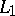is a differential operator of order less than, whose characteristic equation at,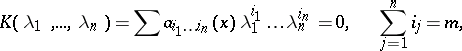has no real roots except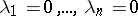.

For second-order equations the characteristic form is quadratic,and can be brought to the form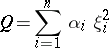by a non-singular affine transformation of the variables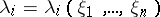,.

When allor all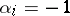, the equation is said to be of elliptic type.

A partial differential equation is said to be of elliptic type in its domain of definition if it is elliptic at every point of this domain.

An elliptic partial differential is called uniformly elliptic if there are positive numbers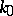and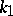such thatFor references see Differential equation, partial.